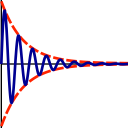Subsections

## spectrum.error_analysis### Synopsis

Perform an error analysis for peak intensities.

### Defaults

spectrum.error_analysis(subset=None)

### Keyword arguments

subset: The list of spectrum ID strings to restrict the error analysis to.

### Description

This user function must only be called after all peak intensities have been loaded and all other necessary spectral information set. This includes the baseplane RMSD and the number of points used in volume integration, both of which are only used if spectra have not been replicated.

The error analysis can be restricted to a subset of the loaded spectral data. This is useful, for example, if half the spectra have been collected on one spectrometer and the other half on a different spectrometer.

Six different types of error analysis are supported depending on whether peak heights or volumes are supplied, whether noise is determined from replicated spectra or the RMSD of the baseplane noise, and whether all spectra or only a subset have been duplicated. These are:

Please see Table 17.25 on page.

 Int type Noise source Error scope Heights RMSD baseplane One sigma per peak per spectrum Heights Partial duplicate + variance averaging One sigma for all peaks, all spectra Heights All replicated + variance averaging One sigma per replicated spectra set Volumes RMSD baseplane One sigma per peak per spectrum Volumes Partial duplicate + variance averaging One sigma for all peaks, all spectra Volumes All replicated + variance averaging One sigma per replicated spectra set

### Peak heights with baseplane noise RMSD

When none of the spectra have been replicated, then the peak height errors are calculated using the RMSD of the baseplane noise, the value of which is set by the spectrum.baseplane_rmsd user function. This results in a different error per peak per spectrum. The standard deviation error measure for the peak height, sigma_I, is set to the RMSD value.

### Peak heights with partially replicated spectra

When spectra are replicated, the variance for a single spin at a single replicated spectra set is calculated by the formula

 sigma^2 = sum({Ii - Iav}^2) / (n - 1),

where sigma^2 is the variance, sigma is the standard deviation, n is the size of the replicated spectra set with i being the corresponding index, Ii is the peak intensity for spectrum i, and Iav is the mean over all spectra i.e. the sum of all peak intensities divided by n.

As the value of n in the above equation is always very low since normally only a couple of spectra are collected per replicated spectra set, the variance of all spins is averaged for a single replicated spectra set. Although this results in all spins having the same error, the accuracy of the error estimate is significantly improved.

If there are in addition to the replicated spectra loaded peak intensities which only consist of a single spectrum, i.e. not all spectra are replicated, then the variances of replicated replicated spectra sets will be averaged. This will be used for the entire experiment so that there will be only a single error value for all spins and for all spectra.

### Peak heights with all spectra replicated

If all spectra are collected in duplicate (triplicate or higher number of spectra are supported), the each replicated spectra set will have its own error estimate. The error for a single peak is calculated as when partially replicated spectra are collected, and these are again averaged to give a single error per replicated spectra set. However as all replicated spectra sets will have their own error estimate, variance averaging across all spectra sets will not be performed.

### Peak volumes with baseplane noise RMSD

The method of error analysis when no spectra have been replicated and peak volumes are used is highly dependent on the integration method. Many methods simply sum the number of points within a fixed region, either a box or oval object. The number of points used, N, must be specified by another user function in this class. Then the error is simply given by the sum of variances:

 sigma_vol^2 = sigma_i^2 * N,

where sigma_vol is the standard deviation of the volume, sigma_i is the standard deviation of a single point assumed to be equal to the RMSD of the baseplane noise, and N is the total number of points used in the summation integration method. For a box integration method, this converts to the Nicholson, Kay, Baldisseri, Arango, Young, Bax, and Torchia (1992) Biochemistry, 31: 5253-5263 equation:

 sigma_vol = sigma_i * sqrt(n*m),

where n and m are the dimensions of the box. Note that a number of programs, for example peakint (http://hugin.ethz.ch/wuthrich/software/xeasy/xeasy_m15.html) does not use all points within the box. And if the number N can not be determined, this category of error analysis is not possible.

Also note that non-point summation methods, for example when line shape fitting is used to determine peak volumes, the equations above cannot be used. Hence again this category of error analysis cannot be used. This is the case for one of the three integration methods used by Sparky (http://www.cgl.ucsf.edu/home/sparky/manual/peaks.html#Integration). And if fancy techniques are used, for example as Cara does to deconvolute overlapping peaks (http://www.cara.ethz.ch/Wiki/Integration), this again makes this error analysis impossible.

### Peak volumes with partially replicated spectra

When peak volumes are measured by any integration method and a few of the spectra are replicated, then the intensity errors are calculated identically as described in the `Peak heights with partially replicated spectra' section above.

### Peak volumes with all spectra replicated

With all spectra replicated and again using any integration methodology, the intensity errors can be calculated as described in the `Peak heights with all spectra replicated' section above.

The relax user manual (PDF), created 2020-08-26.